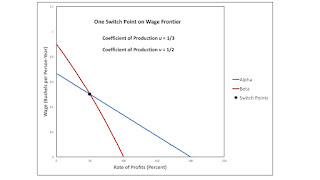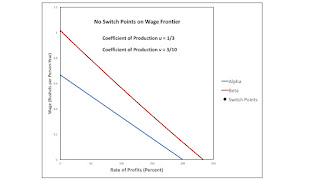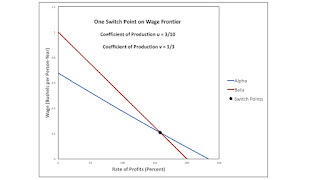## Tuesday, August 01, 2017

### Switch Points Disappearing Or Appearing Over The Axis For The Rate Of ProfitsFigure 1: Two Bifurcation Diagrams Horizontally Reflecting
1.0 Introduction

This post continues my investigation of structural economic dynamics. I am interested in how technological progress can change the analysis of the choice of technique. In this case, I explore how a decrease in a coefficient of production can cause a switch point to appear or disappear over the axis for the rate of profits.

2.0 Technology

Consider the technology illustrated in Table 1. The managers of firms know of four processes of production. And these processes exhibit Constant Returns to Scale. The column for the iron industry specifies the inputs needed to produce a ton of iron. The column for the copper industry likewise specifies the inputs needed to produce a ton of copper. Two processes are known for producing corn, and their coefficients of production are specified in the last two columns in the table. Each process is assumed to require a year to complete and uses up all of its commodity inputs. Technology is defined in terms of two parameters, u and v. u denotes the quantity of iron needed to produce a unit iron in the iron industry. v is the quantity of copper needed to produce a unit copper.

 Input Industry Iron Copper Corn Alpha Beta Labor 1 2/3 1 2/3 Iron u 0 1/3 0 Copper 0 v 0 1/3 Corn 0 0 0 0

As usual, this technology presents a problem of the choice of technique. The Alpha technique consists of the iron-producing process and the corn-producing process labeled Alpha. Similarly, the Beta technique consists of the copper-producing process and the corn-producing process labeled Beta.

I make all my standard assumptions. The choice of technique is based on cost-minimization. Consider prices of production, which are stationary prices that allow the economy to be reproduced. A wage curve, showing a tradeoff between wages and the rate of profits, is associated with each technique. In drawing such a curve, I assume that a bushel corn is the numeraire and that labor is advanced. Hence, wages are paid out of the surplus product at the end of the year. The chosen technique, for, say, a given wage, maximizes the rate of profits. The wage curve for the cost-minizing technique at the given wage lies on the outer frontier formed from all wage curves.

3.0 Innovations

I have two stories of technical innovation. In one, improvements are made in the process for producing copper. As a consequence, the wage curve for the Beta technique moves outward. In the other story, improvements are made in the iron industry, and the wage curve for the Alpha technique moves outwards. The bifurcations that occur in the two stories are mirror reflections of one another, in some sense.

3.1 Improvements in Copper Production

Let u be fixed at 1/3 tons per ton. The wage curve for the Alpha technique is a downward sloping straight line. Let v decrease from 1/2 to 3/10. When v is 1/3, the wage curve for the Beta technique is also a straight line. I created the example to have linear (actually, affine) wage curves at the bifurcation for convenience. The bifurcation does not require such.

Figure 2 shows the wage curves when the copper coefficient for copper production is a high value, in the range under consideration. A single switch point exists, and the Alpha technique is cost-minimizing if the rate of profits is high. As v decreases, the switch point moves to a higher and higher rate of profits. (These statements are about the shapes of mathematical functions. They are not about historical processes set in time.) Figure 3 shows the wage curves when v is 1/3. The switch point is now on the axis for the rate of profits. For any non-negative rate of profits below the maximum, the Beta technique is cost-minimizing. Finally, Figure 4 shows the wage curves for an even lower copper coefficient in copper production. Now, there is no switch point, and the Beta technique is always cost-minimizing, for all possible prices of production.Figure 2: Wage Curves Without Improvement in Copper ProductionFigure 3: Wage Curves For A BifurcationFigure 4: Wage Curves After Improvements in Copper Production

3.2 Improvements in Iron Production

Now let v be set at 1/3. Let u decrease from 1/2 to 3/10. Figure 5 shows the wage curves at the high end for the iron coefficient in iron production. No switch point exists, and the Beta technique is always cost-minimizing. I thought about repeating Figure 3, for v decreased to 1/3. The same configuration of wage curves, with a bifurcation, appears in this story. Figure 6, shows that the switch point appears for an even lower value of the iron coefficient.Figure 5: Wage Curves Without Improvement in Iron ProductionFigure 6: Wage Curves After Improvements in Iron Production

3.3 Improvements in Both Iron and Copper Industries

I might as well graph (Figure 7) the copper coefficient in copper production against the iron coefficient in iron production. The bifurcation occurs when the maximum rates of profits are identical in the Alpha and Beta technique. In a model with the simple structure of the example, this occurs when u = v. Representative illustrations of wage curves are shown in the regions in the parameter space. A switch point below the maximum rate of profits exists only above the line in parameter space representing the bifurcation.Figure 7: Bifurcation Diagram for Two Coefficients of Production

The story in Section 3.1 corresponds to moving downwards on a vertical line in Figure 7. The left-hand side of Figure 1, at the top of this post, is another way of illustrating this story. On the other hand, Section 3.2 tells a story of moving leftwards on a horizontal line in Figure 7. The right-hand side of Figure 1 illustrates this story.

Focus on the intersections, in the two sides of Figure 1 of the blue, red, and purple loci. Can you see that, in some sense, they are reflections, up to a topological equivalence?

4.0 Discussion

I have a reswitching example with a switch point disappearing over the axis for the rate of profits. In that example, the disappearing switch point is 'perverse', that is, it has a positive real Wicksell effect. In the examples in Section 3 above, the disappearing or appearing switch point is 'normal', with a negative real Wicksell effect.